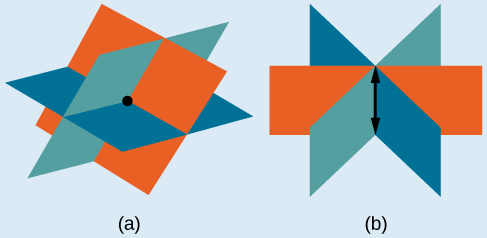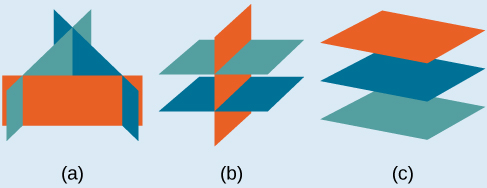# 11.2 Systems of linear equations: three variables

 Page 1 / 8
In this section, you will:
• Solve systems of three equations in three variables.
• Identify inconsistent systems of equations containing three variables.
• Express the solution of a system of dependent equations containing three variables.

John received an inheritance of $12,000 that he divided into three parts and invested in three ways: in a money-market fund paying 3% annual interest; in municipal bonds paying 4% annual interest; and in mutual funds paying 7% annual interest. John invested$4,000 more in municipal funds than in municipal bonds. He earned \$670 in interest the first year. How much did John invest in each type of fund?

Understanding the correct approach to setting up problems such as this one makes finding a solution a matter of following a pattern. We will solve this and similar problems involving three equations and three variables in this section. Doing so uses similar techniques as those used to solve systems of two equations in two variables. However, finding solutions to systems of three equations requires a bit more organization and a touch of visual gymnastics.

## Solving systems of three equations in three variables

In order to solve systems of equations in three variables, known as three-by-three systems, the primary tool we will be using is called Gaussian elimination , named after the prolific German mathematician Karl Friedrich Gauss . While there is no definitive order in which operations are to be performed, there are specific guidelines as to what type of moves can be made. We may number the equations to keep track of the steps we apply. The goal is to eliminate one variable at a time to achieve upper triangular form , the ideal form for a three-by-three system because it allows for straightforward back-substitution to find a solution $\text{\hspace{0.17em}}\left(x,y,z\right),\text{}$ which we call an ordered triple . A system in upper triangular form looks like the following:

The third equation can be solved for $\text{\hspace{0.17em}}z,\text{}$ and then we back-substitute to find $\text{\hspace{0.17em}}y\text{\hspace{0.17em}}$ and $\text{\hspace{0.17em}}x.\text{\hspace{0.17em}}$ To write the system in upper triangular form, we can perform the following operations:

1. Interchange the order of any two equations.
2. Multiply both sides of an equation by a nonzero constant.
3. Add a nonzero multiple of one equation to another equation.

The solution set to a three-by-three system is an ordered triple $\text{\hspace{0.17em}}\left\{\left(x,y,z\right)\right\}.\text{\hspace{0.17em}}$ Graphically, the ordered triple defines the point that is the intersection of three planes in space. You can visualize such an intersection by imagining any corner in a rectangular room. A corner is defined by three planes: two adjoining walls and the floor (or ceiling). Any point where two walls and the floor meet represents the intersection of three planes.

## Number of possible solutions

• Systems that have a single solution are those which, after elimination, result in a solution set    consisting of an ordered triple $\text{\hspace{0.17em}}\left\{\left(x,y,z\right)\right\}.\text{\hspace{0.17em}}$ Graphically, the ordered triple defines a point that is the intersection of three planes in space.
• Systems that have an infinite number of solutions are those which, after elimination, result in an expression that is always true, such as $\text{\hspace{0.17em}}0=0.\text{\hspace{0.17em}}$ Graphically, an infinite number of solutions represents a line or coincident plane that serves as the intersection of three planes in space.
• Systems that have no solution are those that, after elimination, result in a statement that is a contradiction, such as $\text{\hspace{0.17em}}3=0.\text{\hspace{0.17em}}$ Graphically, a system with no solution is represented by three planes with no point in common.(a)Three planes intersect at a single point, representing a three-by-three system with a single solution. (b) Three planes intersect in a line, representing a three-by-three system with infinite solutions.All three figures represent three-by-three systems with no solution. (a) The three planes intersect with each other, but not at a common point. (b) Two of the planes are parallel and intersect with the third plane, but not with each other. (c) All three planes are parallel, so there is no point of intersection.

A laser rangefinder is locked on a comet approaching Earth. The distance g(x), in kilometers, of the comet after x days, for x in the interval 0 to 30 days, is given by g(x)=250,000csc(π30x). Graph g(x) on the interval [0, 35]. Evaluate g(5)  and interpret the information. What is the minimum distance between the comet and Earth? When does this occur? To which constant in the equation does this correspond? Find and discuss the meaning of any vertical asymptotes.
The sequence is {1,-1,1-1.....} has
how can we solve this problem
Sin(A+B) = sinBcosA+cosBsinA
Prove it
Eseka
Eseka
hi
Joel
June needs 45 gallons of punch. 2 different coolers. Bigger cooler is 5 times as large as smaller cooler. How many gallons in each cooler?
7.5 and 37.5
Nando
find the sum of 28th term of the AP 3+10+17+---------
I think you should say "28 terms" instead of "28th term"
Vedant
the 28th term is 175
Nando
192
Kenneth
if sequence sn is a such that sn>0 for all n and lim sn=0than prove that lim (s1 s2............ sn) ke hole power n =n
write down the polynomial function with root 1/3,2,-3 with solution
if A and B are subspaces of V prove that (A+B)/B=A/(A-B)
write down the value of each of the following in surd form a)cos(-65°) b)sin(-180°)c)tan(225°)d)tan(135°)
Prove that (sinA/1-cosA - 1-cosA/sinA) (cosA/1-sinA - 1-sinA/cosA) = 4
what is the answer to dividing negative index
In a triangle ABC prove that. (b+c)cosA+(c+a)cosB+(a+b)cisC=a+b+c.
give me the waec 2019 questionsByByByBy